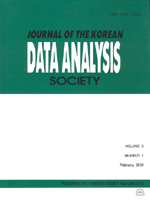상세검색
최근 검색어 전체 삭제
다국어입력
즐겨찾기0KCI등재 학술저널

# Asymptotic Overfitting Probabilities of some Model Selection Criteria in Large Samples

• 등재여부 : KCI등재
• 2007.06
• 1037 - 1048 (12 pages)

We look at overfitting in large samples by obtaining asymptotic probabilities of some model selection criteria, which are AIC, AICc, AICu, SIC, HQ, HQc, Cp, FPE, and FPEu. Asymptotic Overfitting probabilities refer to comparing one overfitted model of order k_{*} +L to the reduced model of order k_{*}. We make use of the following fact : As n rarrow INF and where k_{*} and L are fixed, {chi_{ n-k_{*} -L}^{2}} over { n-k_{*} -L} rarrow 1. It is clear that AIC, AICc, FPE and Cp are not constant criteria, since their probabilities of overfitting are not 0. But SIC, HQ, and HQc are consistent and thus do have 0 probability of overfitting.

1. Introduction

2. Review of model selection criteria

3. Asymptotic Probabilities of Overfitting

4. Conclusions

References